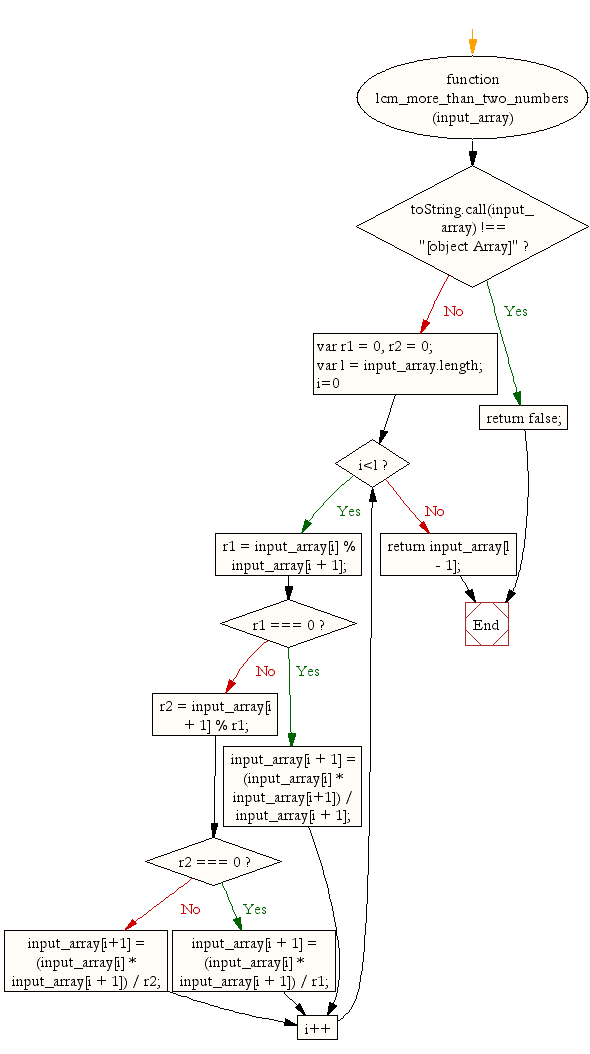# JavaScript: Least common multiple (LCM) of more than 2 integers

## JavaScript Math: Exercise-11 with Solution

Write a JavaScript function to get the least common multiple (LCM) of more than 2 integers.

Test Data :
console.log(lcm_more_than_two_numbers([100,90,80,7]));
console.log(lcm_more_than_two_numbers([5,10,15,25]));
Output :
25200
150

Sample Solution:-

HTML Code:

``````<!DOCTYPE html>
<html>
<meta charset="utf-8">
<title>LCM more than two numbers</title>
<body>
</body>
</html>
```
```

JavaScript Code:

``````function lcm_more_than_two_numbers(input_array) {
if (toString.call(input_array) !== "[object Array]")
return  false;
var r1 = 0, r2 = 0;
var l = input_array.length;
for(i=0;i<l;i++) {
r1 = input_array[i] % input_array[i + 1];
if(r1 === 0) {
input_array[i + 1] = (input_array[i] * input_array[i+1]) / input_array[i + 1];
}
else {
r2 = input_array[i + 1] % r1;
if(r2 === 0) {
input_array[i + 1] = (input_array[i] * input_array[i + 1]) / r1;
}
else {
input_array[i+1] = (input_array[i] * input_array[i + 1]) / r2;
}
}
}
return input_array[l - 1];
}

console.log(lcm_more_than_two_numbers([100,90,80,7]));
console.log(lcm_more_than_two_numbers([5,10,15,25]));
```
```

Sample Output:

```25200
150
```

Flowchart:Live Demo:

See the Pen javascript-math-exercise-11 by w3resource (@w3resource) on CodePen.

Improve this sample solution and post your code through Disqus

What is the difficulty level of this exercise?

Test your Programming skills with w3resource's quiz.

﻿